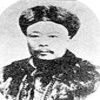#### You may also like### Very Old Man

Is the age of this very old man statistically believable?### Reaction Timer Timer

How can you time the reaction timer?### Robin's Hypothesis Testing

How many trials should we do in order to accept or reject our null hypothesis?

# Chi-squared Faker

##### Age 16 to 18 Challenge Level:

The $\chi^2$ test statistic is given by
$$\chi^2 = \sum\frac{(f_o-f_e)^2}{f_e}$$

The weights of a certain type of primate are known through extensive studies to take an expected distribution, given by the expected value below. The weights of a community of these primates from a different location are measured, and are listed in the observed values below:

 Weight (kg) [0, 9] [10,19] [20,29] [30,39] [40,49] [50,59] Expected 3 3 3 4 8 9 Observed 5 6 3 5 7 7

 Weight (kg) [60,69] [70,79] [80,89] [90,99] [100,109] [110,119] [120+] Expected 11 12 8 10 4 12 13 Observed 12 17 7 2 12 16 15

How would you describe the expected distribution? Can you think of a good explanation for this pattern of expected data?

You are asked to undertake a Chi-squared test to assess the hypothesis that the weights of the two populations are driven by the same distribution.

Supposing that for unscientific reasons you were keen on rejecting the hypothesis. Before making any detailed calculations, what would be the best way to proceed with the Chi-squared test to make this happen?

Conversely, how might you organise your calculation to maximise the chance of accepting the hypothesis? If you can think of several ways in which to do this, which seems most natural?

Perform the tests to see if you were correct.

Do you think that the data should be accepted or rejected at the 1% significance level?

NOTES AND BACKGROUND

As Benjamin Disraeli famously said, 'There are lies, damned lies and statistics'. This problem shows that the notion of 'significance' is not necessarily as clearly cut as the layman might imagine: data can often easily be manipulated to present a variety of possibly misleading pictures. Sometimes this manipulation is purposeful and sometimes due to 'blind' application of an algorithm. Trained statisticians often reserve a sceptical eye when presented with the results of significance tests and are always aware of the assumptions going into a calculation and the implications of these.